maths > wholenumbers

Large Numbers

what you'll learn...

overview

In this page, the large numbers from 10$10$ to 1000$1000$ are introduced with the following important concepts.

•  10$10$ units grouped to 1$1$ ten.

•  representation of numbers with units, tens, hundreds, thousands, etc.

This forms the foundation to understanding grouping, de-grouping, regrouping used in numerical arithmetics.

Grouping

•  grouping of 10$10$ of units to 1$1$ ten

•  introduces the basics to representing numbers in place-value.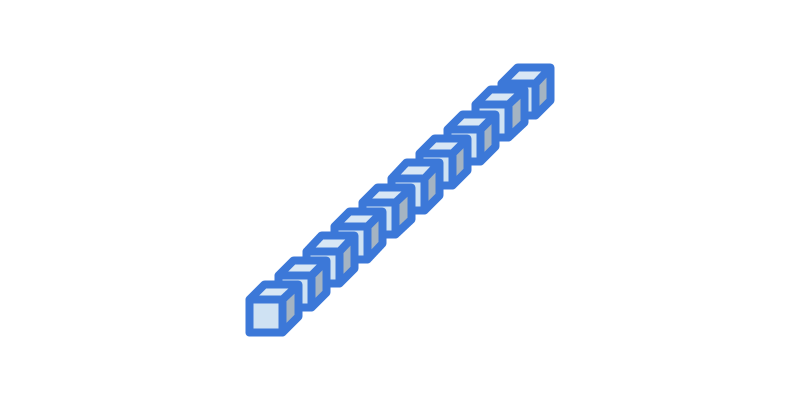There are 10$10$ blue colored blocks in the picture.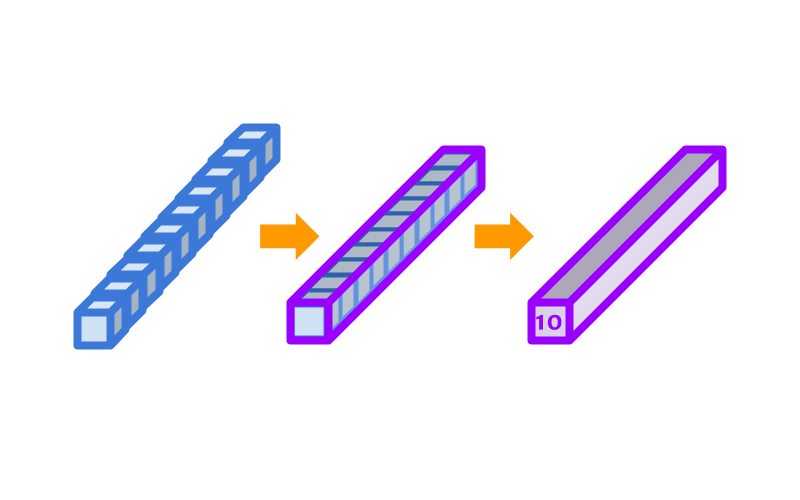There are $10$ blue color blocks in the picture. These blocks are packed into a purple block and marked $10$. In this course, the purple block is used to represent $10$.

Blue block represents $1$ and the grouped purple block represent $10$.
Consider that the smaller blue block is a lower place-value and the larger purple block is higher place-value.

Combining a $10$ of lower place value into $1$ of higher place value is grouping.

The word grouping means joining and combining together. The blocks were combined into one bigger block.

Grouping: $10$ of a lower place value is combined to $1$ of higher place value.

Numbers above 10The number represented in the figure is $11$. The purple block is used to represent ten and additional block represents $1$. So, the total is $11$.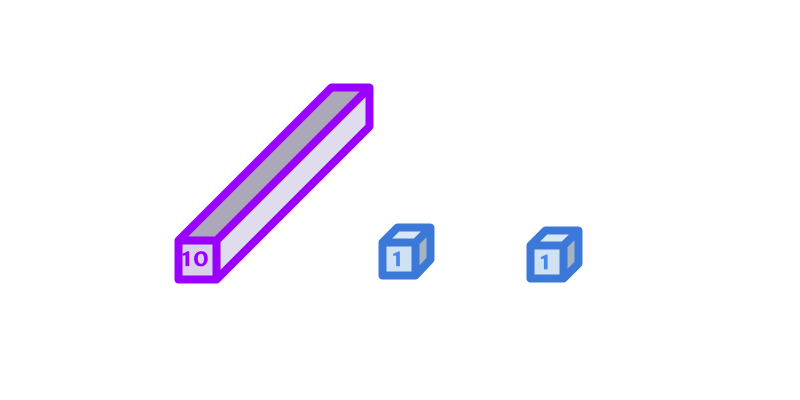The number represented in the figure is $12$.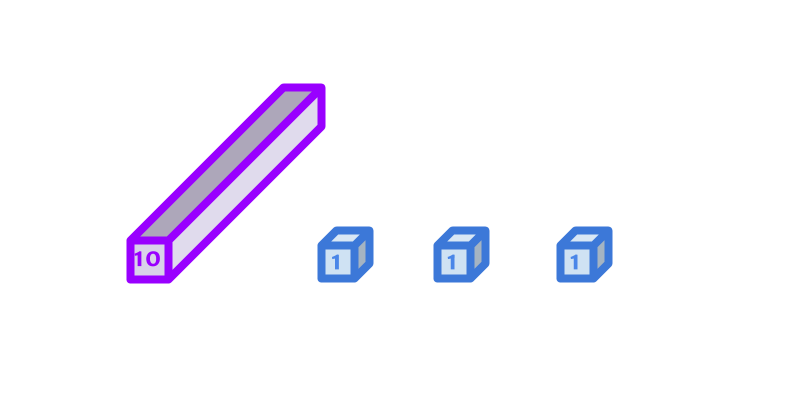The number represented in the figure is $13$.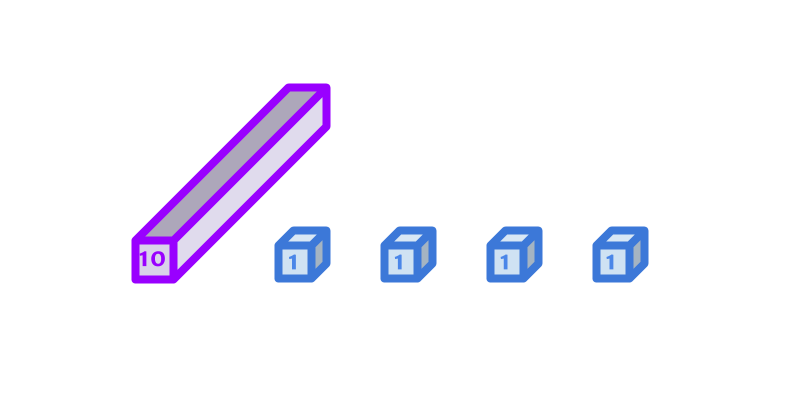The number represented in the figure is $14$.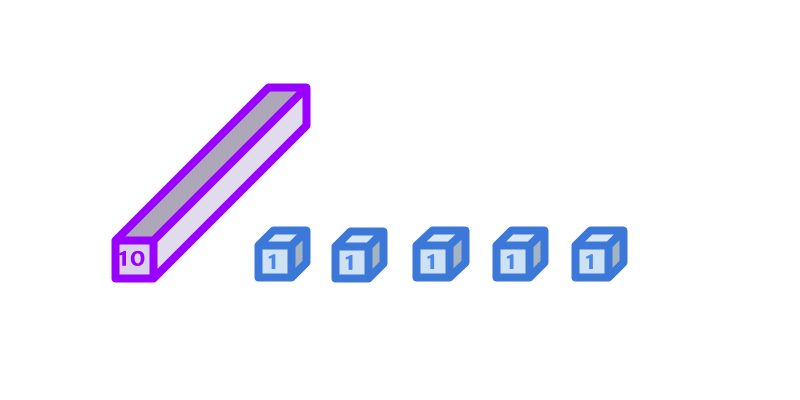The number represented in the figure is $15$.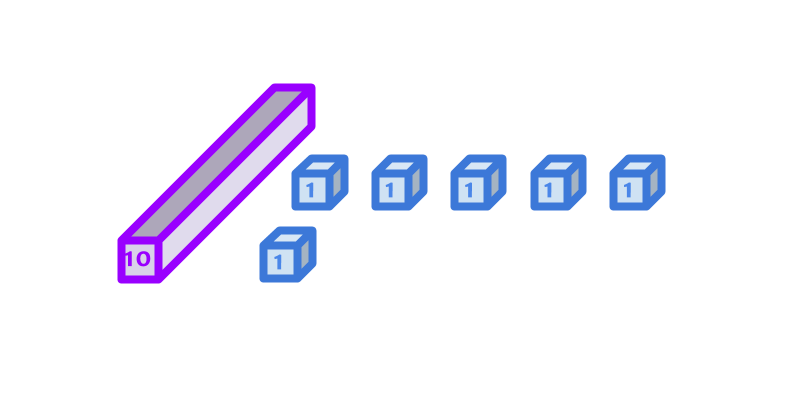The number represented in the figure is $16$.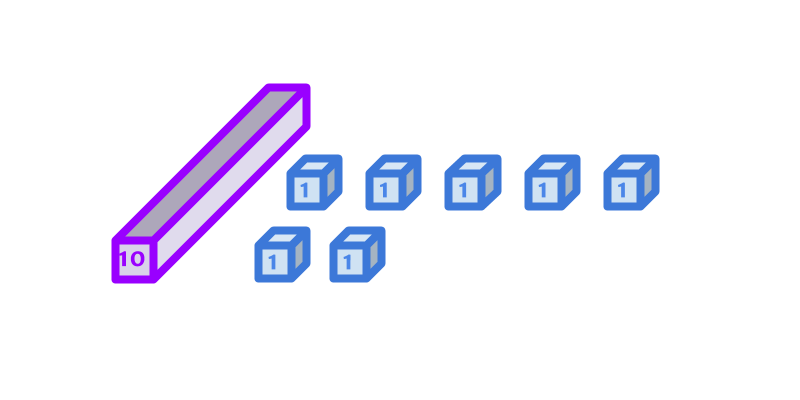The number represented in the figure is $17$.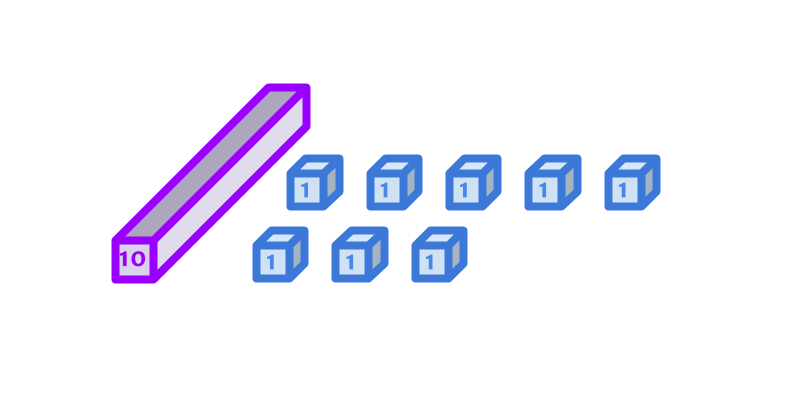The number represented in the figure is $18$.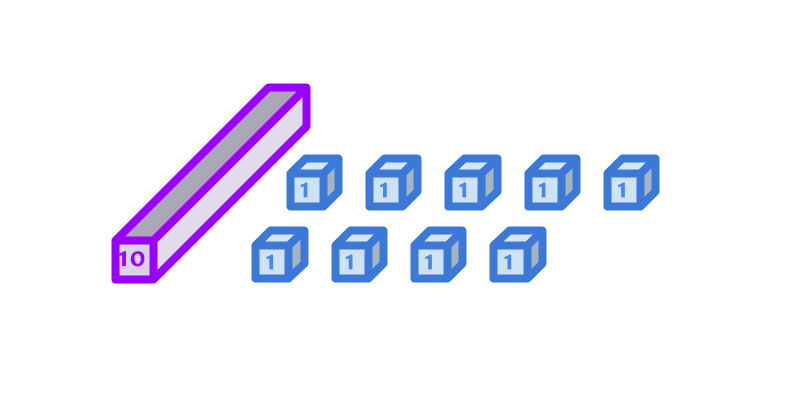The number represented in the figure is $19$.

Numbers above $10$: The numbers above 10 are
$11$ (eleven),
$12$ (twelve),
$13$ (thirteen),
$14$ (fourteen),
$15$ (fifteen),
$16$ (sixteen),
$17$ (seventeen),
$18$ (eighteen),
$19$ (nineteen).

tens and units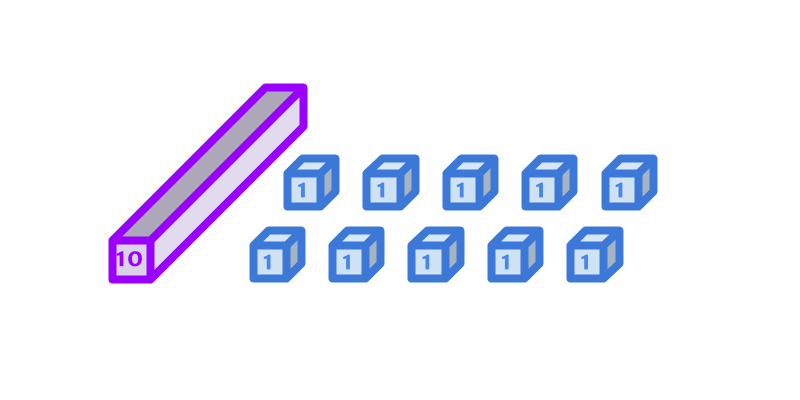The number represented in the figure is $20$.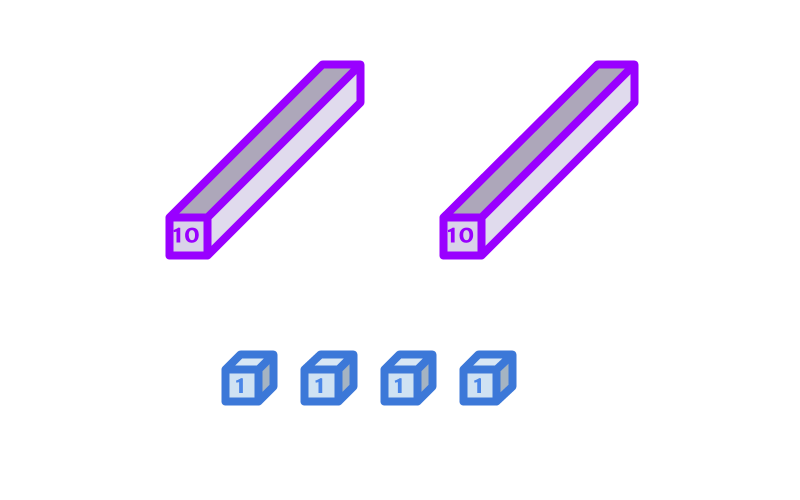The number represented in the figure is $24$.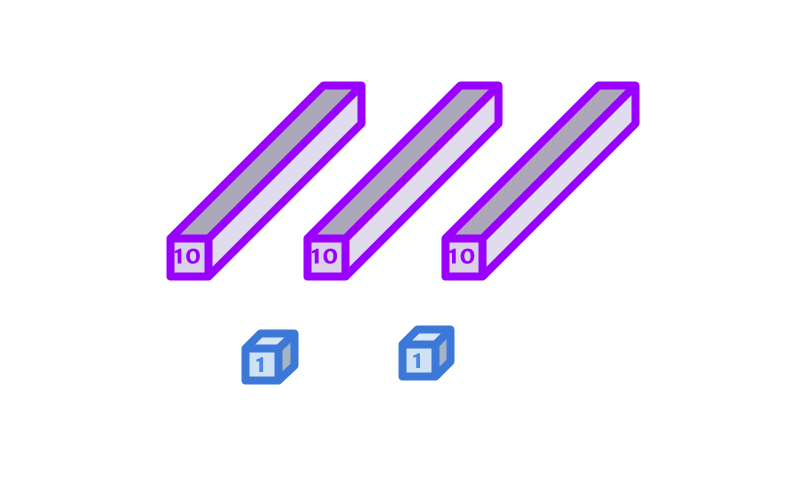The number represented in the figure is $32$.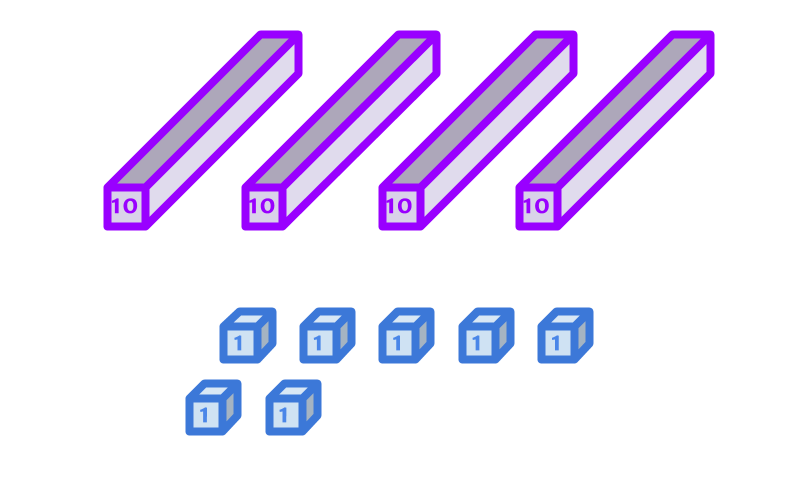The number represented in the figure is $47$.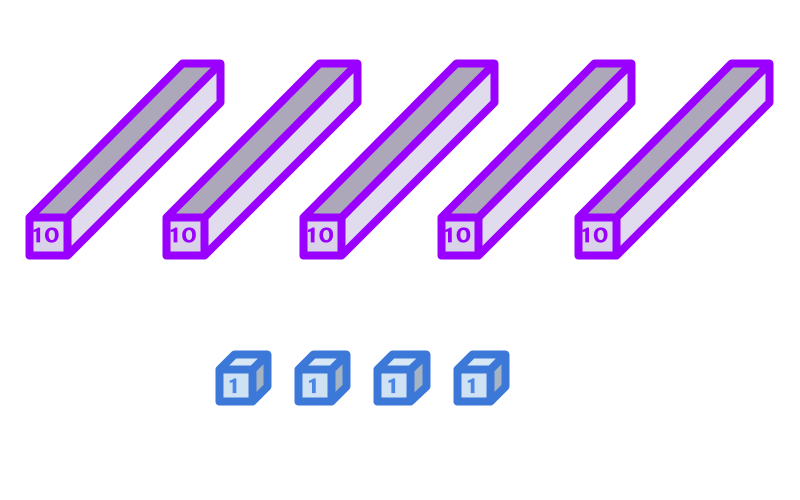The number represented in the figure is $54$.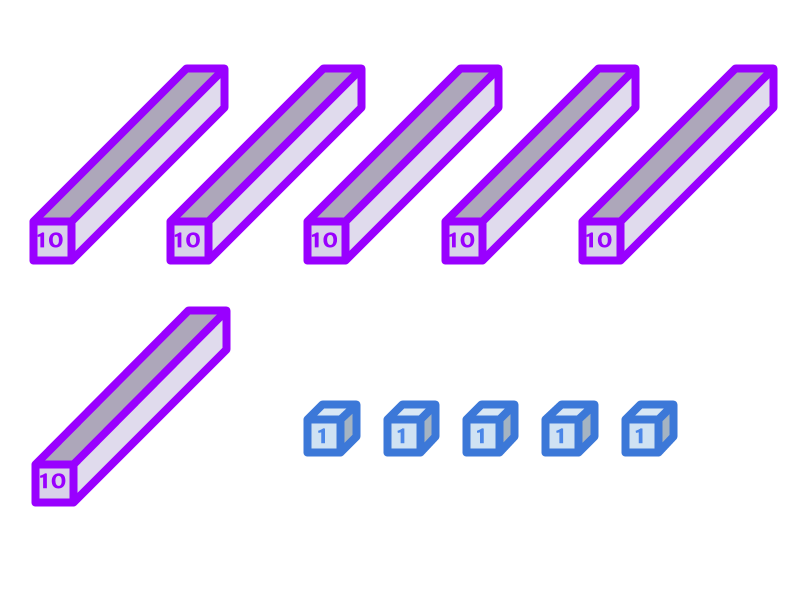The number represented in the figure is $65$.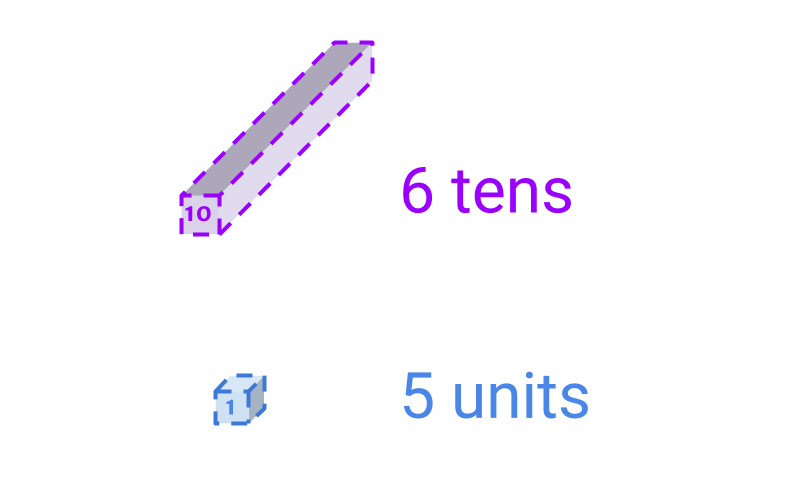The number represented in the figure is $65$.

Instead of laying out all the blocks, the representation gives the count of each of the block. The blocks are of sizes $1$, and $10$.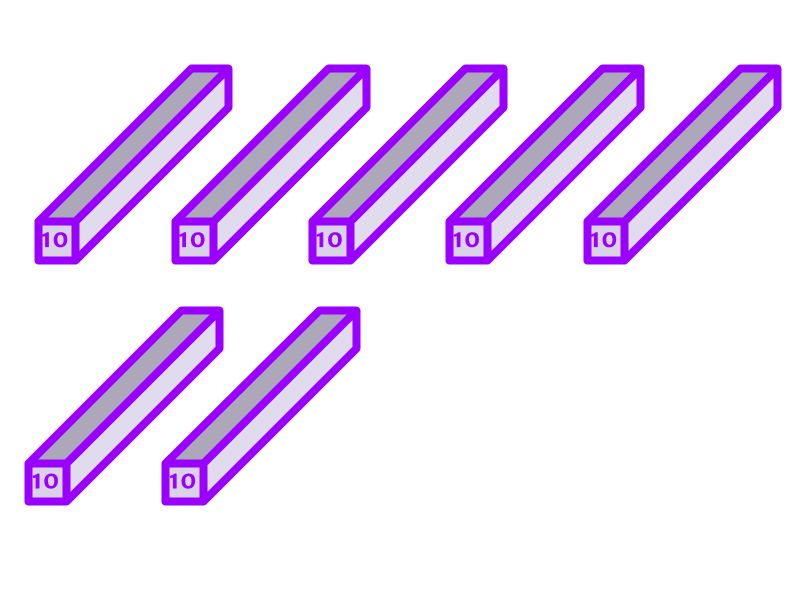The number represented in the figure is $70$.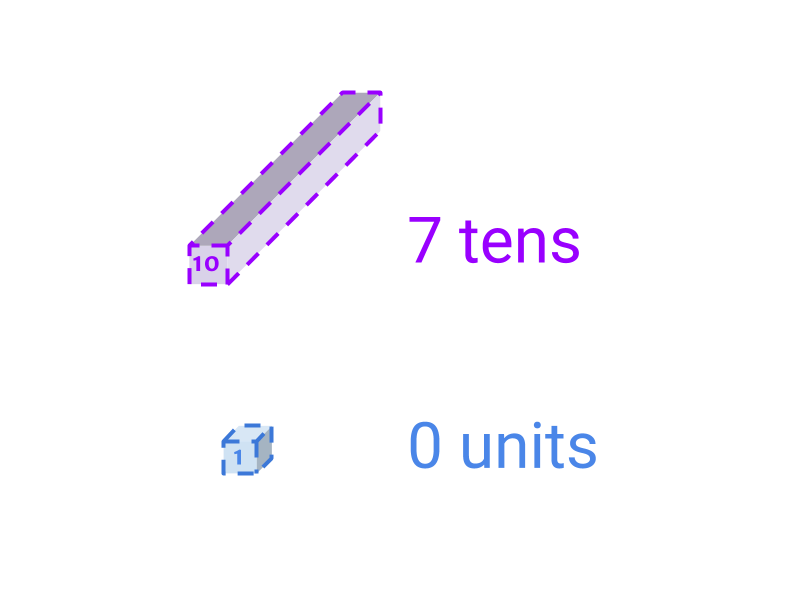The picture provides an equivalent form of representing $70$.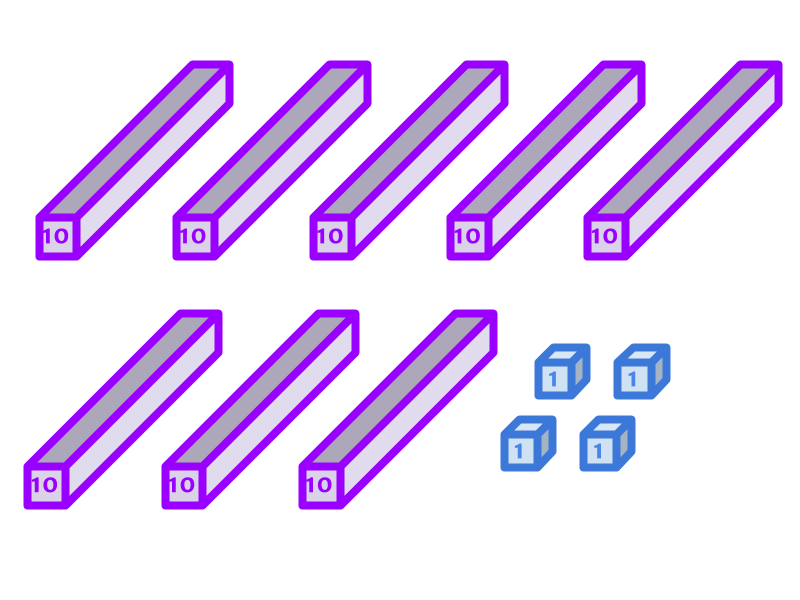The number represented in the figure is $84$.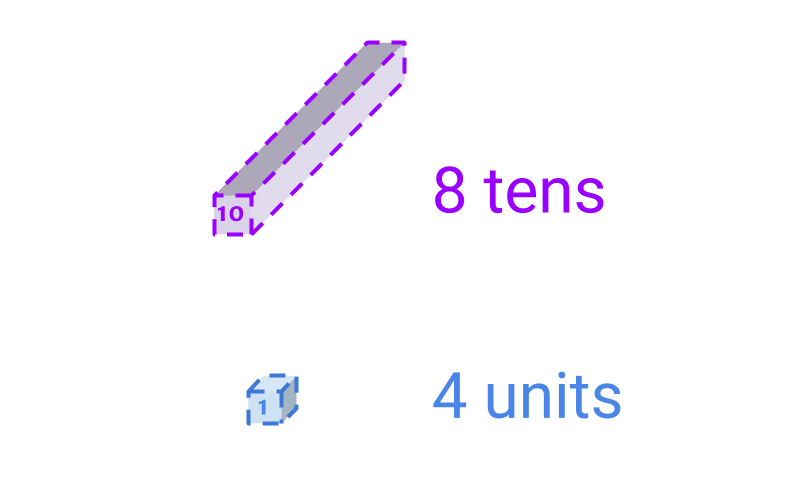The picture provides an equivalent form of representing $84$.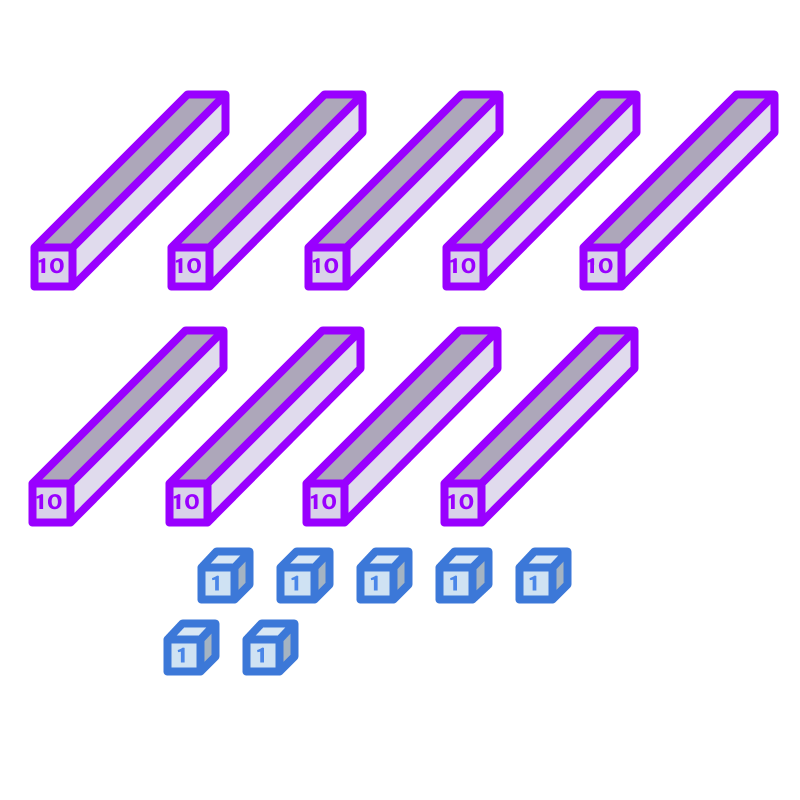The number represented in the figure is $97$.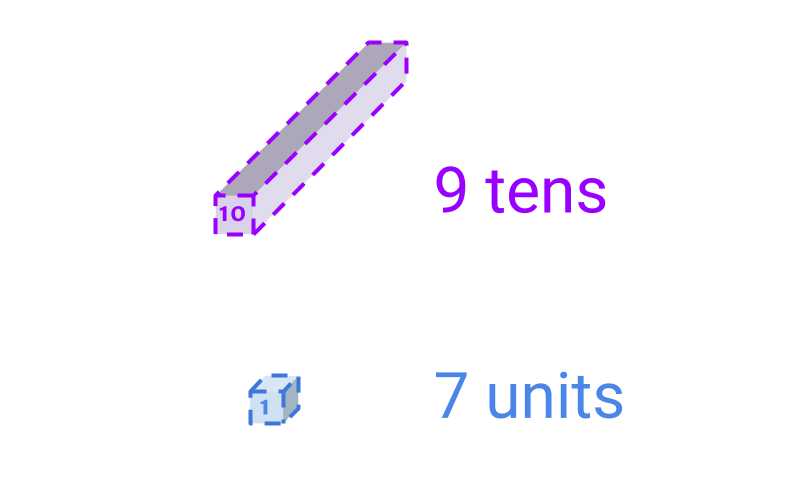The picture provides an equivalent form of representing $97$.

Numbers in the tens:
$10$ (ten),
$20$ (twenty),
$30$ (thirty),
$40$ (forty),
$50$ (fifty),
$60$ (sixty),
$70$ (seventy),
$80$ (eighty),
$90$ (ninety)

Hundreds and Large Numbers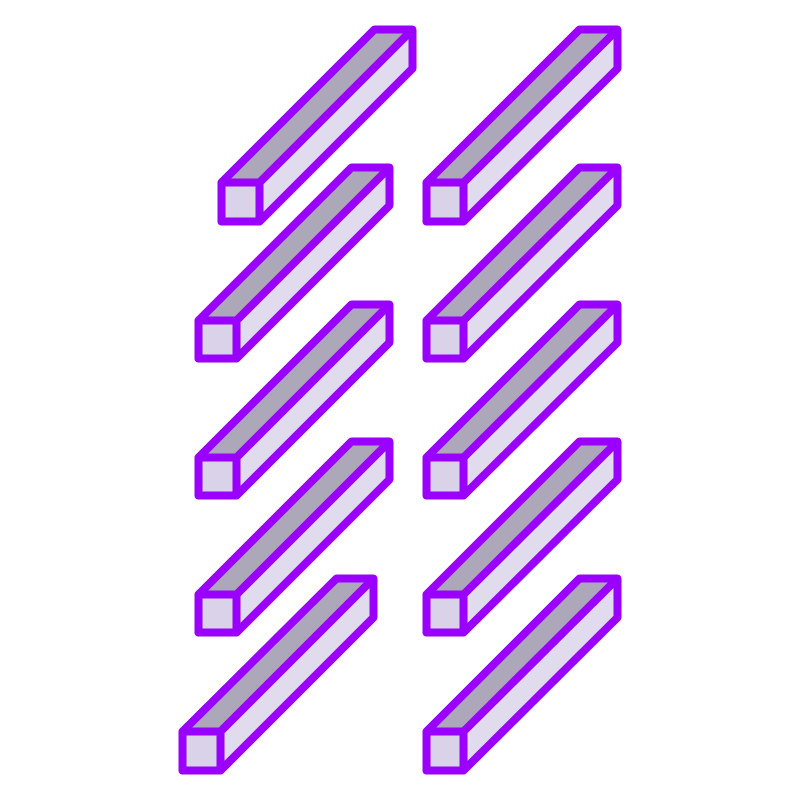The number represented in the figure is $100$.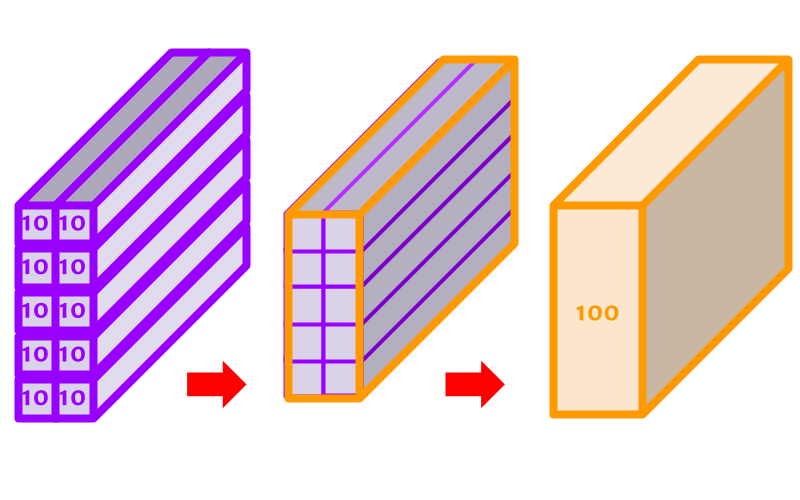Note that $10$ of purple blocks are grouped into an orange block. The orange block represents $100$.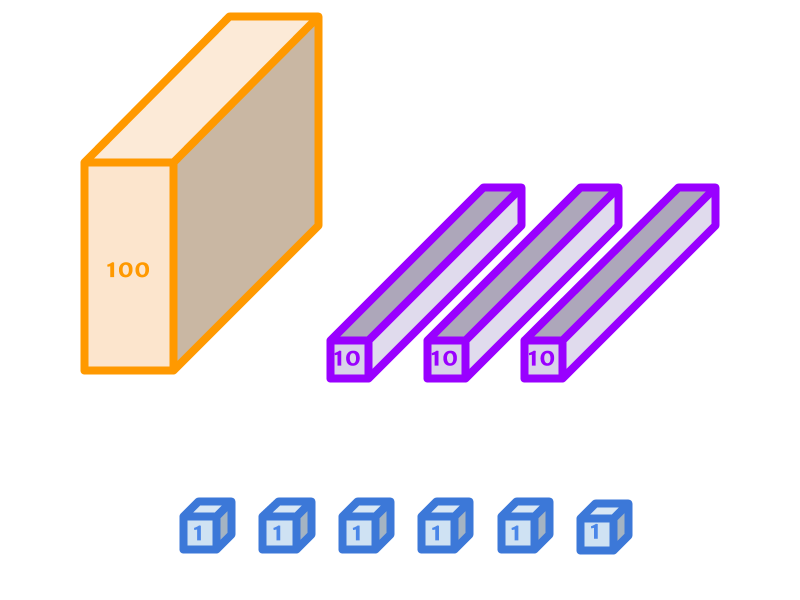The number represented in the figure is $136$.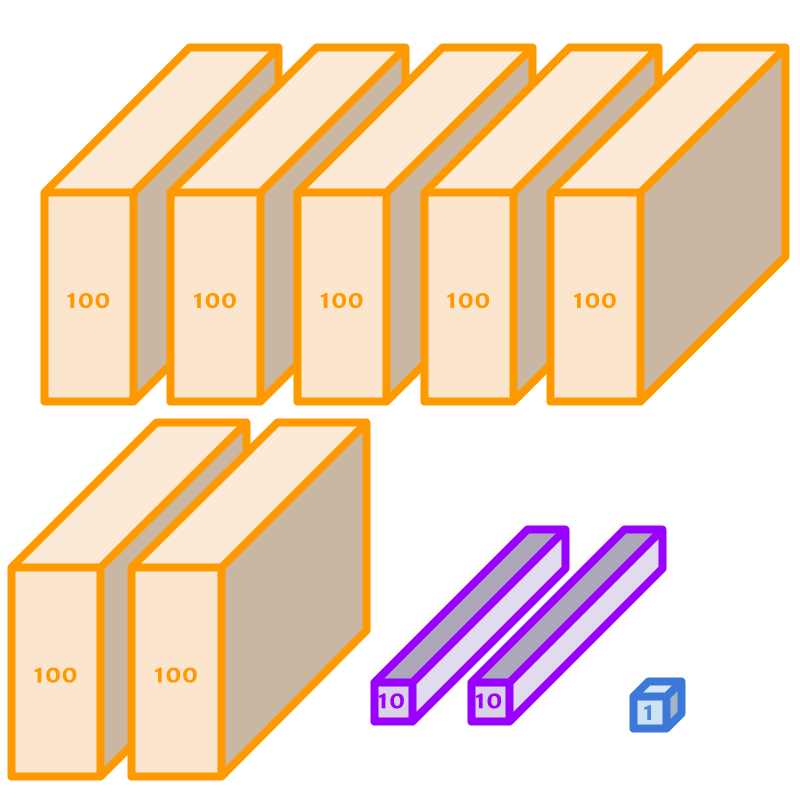The number represented in the figure is $721$.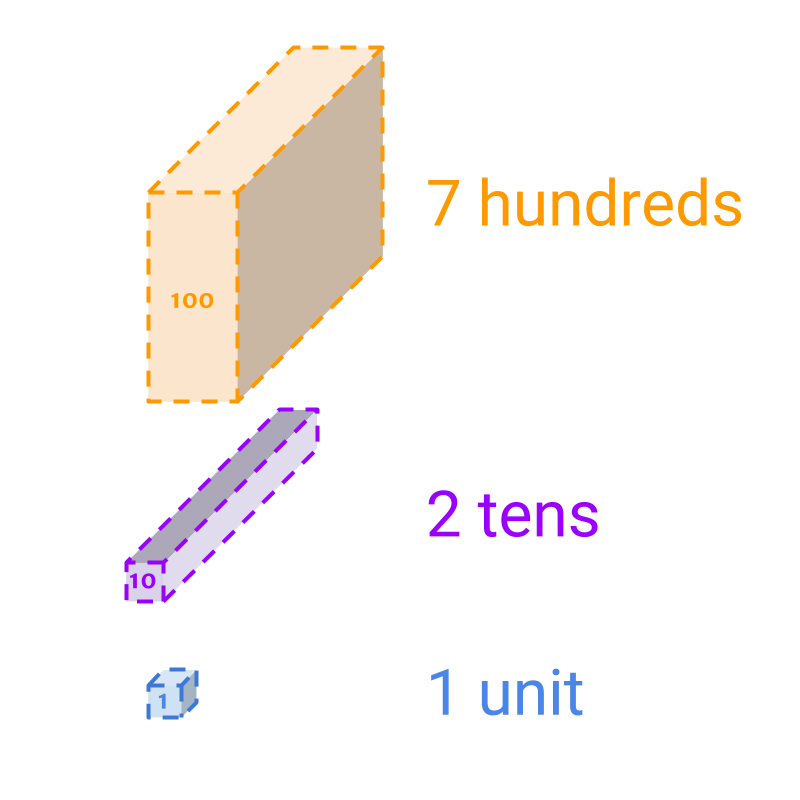The number represented in the figure is $721$. This provides a simpler form.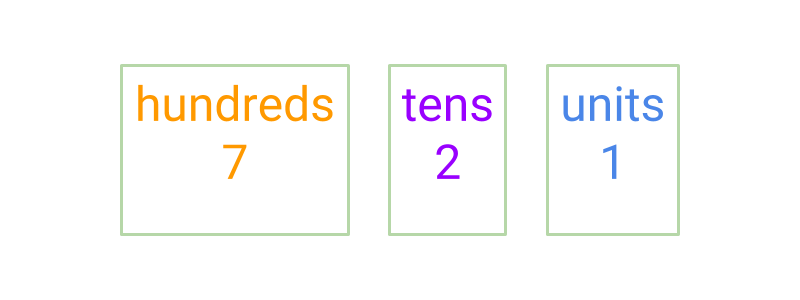The number represented in the figure is $721$. Instead of providing a pictorial representation of the blocks, the value is given in words. The values are hundreds, tens, and units, equivalently representing blocks of size $100$, $10$, and $1$.

Numbers are represented as sequence of digits. If $721$ is given, the number of digits are counted as $3$ and the number is read as seven hundred and twenty one.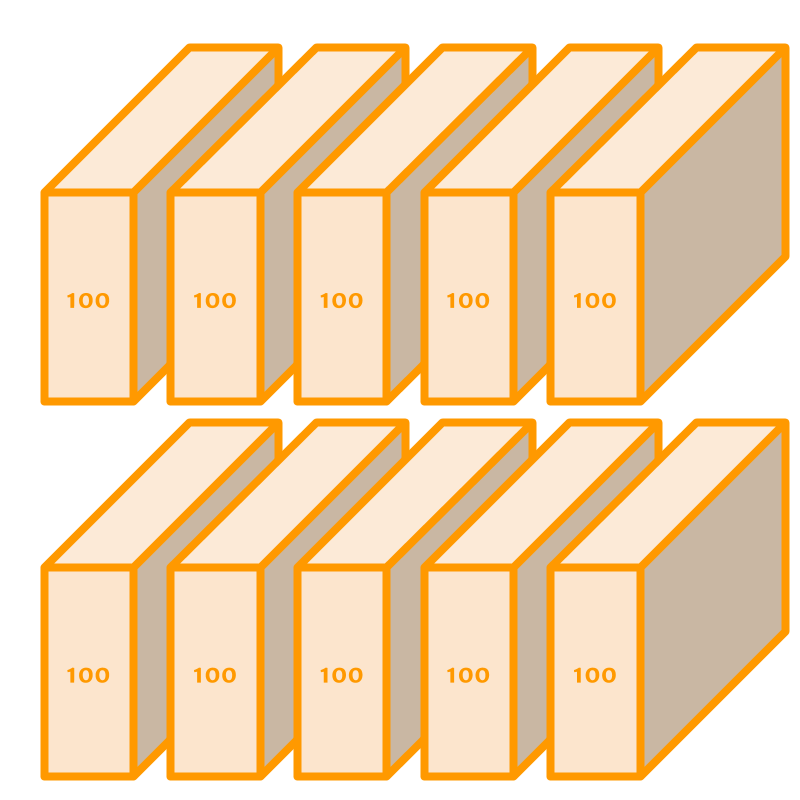The number represented in the figure is $1000$.

$1000$ is written as thousand.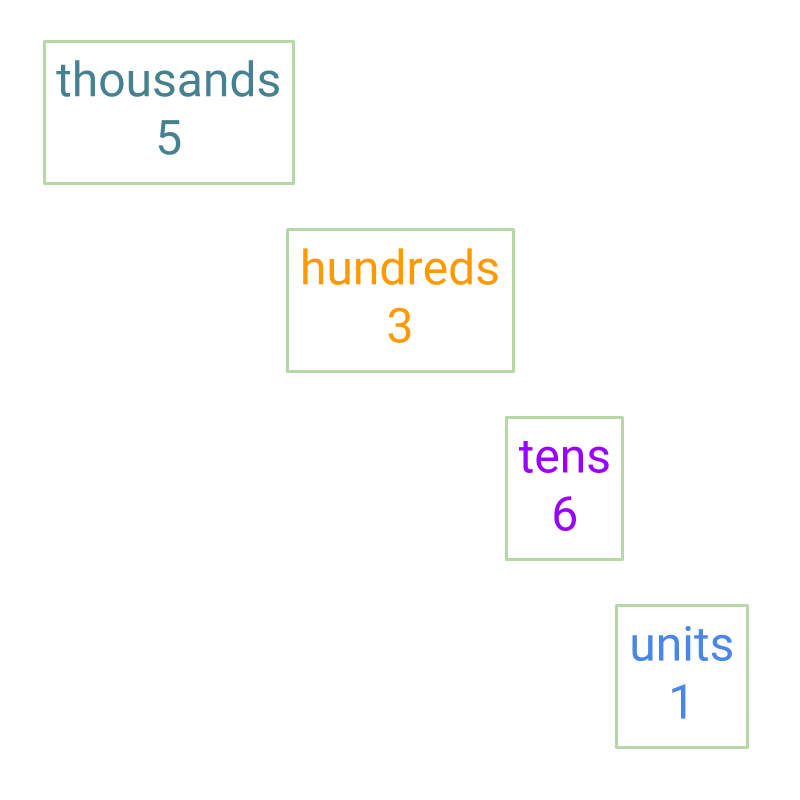The number represented in the figure is $5361$.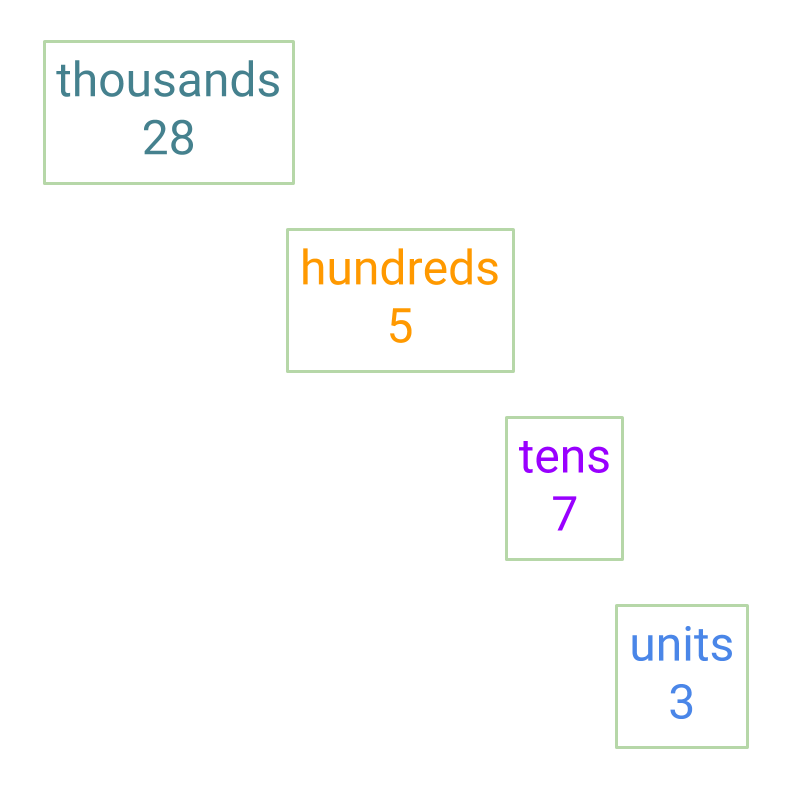The number represented in the picture is $28573$

Summary

The units, tens, hundreds, thousands, etc are explained and numbers upto $10000$ are introduced.The figure shows grouping of $10$ units to $1$ ten. This grouping is reversible and de-grouped when required. And, units can be regrouped into tens.

Grouping: $10$ of a lower place value is combined to $1$ of higher place value.The figure shows the representation of $84$ in blocks.The figure shows number $84$ in abstracted form $8$ tens and $4$ units.The figure shows grouping of $10$ tens to $1$ hundred.The figure shows the representation of $721$ in blocks.The figure shows the representation of $721$ in hundreds, tens, units format.The figure shows the representation of $721$ in number format.The figure shows $5361$ in numbers. Large Numbers : The digits in large numbers are

$1$ (units),
$10$ (tens),
$100$ (hundreds),

$1 , 000$ (thousands)
$10 , 000$ (ten thousands)

Outline